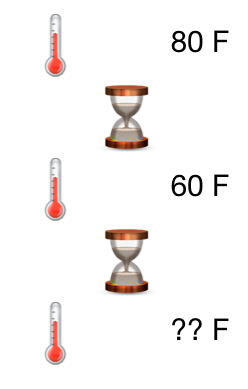# Newton's Law Of CoolingA thermometer reading $80^\circ F$ is taken outside.
Five minutes later the thermometer reads $60^\circ F$.
After another 5 minutes it reads $50^\circ F$.

What is the temperature outside $($in $^\circ F)?$

Assume that this process follows Newton's law of cooling.

×